# Area Volume Perimeter Surface Area Formulas PDF + Printable#Area Perimeter Volume and Surface Area Formulas

An online geometry formulas in pdf format.

## Angles

A right angle is made up of 90 degrees.A straight line is made up of 180 degrees.If two lines intersect, the sum of the resulting four angles equals 360.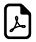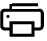Geometric FigureAreaPerimeter
Area of a Parallelogram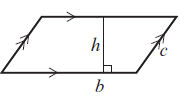A = b x hP =2(b + c)
Area of a Triangle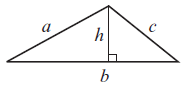P = a + b + c
Area of a Trapezium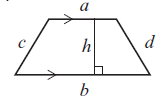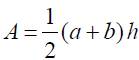P = a + b + c + d
Area of a CircleA = πr2C = 2πr
Area of a Rectangle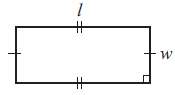A = l x wP = 2(l + w)# Volume and Perimeter Surface Area FormulasGeometric FigureVolumeSurface Area
Volume of a Pyramid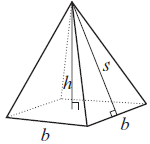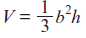A = 2bs + b2
Volume of a Cuboid / Rectangular prismV = lwhA = 2(wh + lw + lh)
Volume of a Cylinder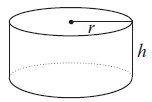V = πr2hA = 2πr2 + 2πrh
Volume of a Sphere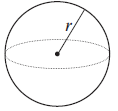A = 4πr2
Volume of a Cone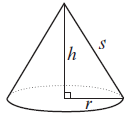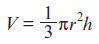A = πrs + πr2
Volume of a Triangular Prism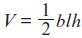A = ah + bh + ch + bl##Age Calculators ►

Horse Age Calculator , Cow Age Calculator , Cat Age Calculator , Dog Age Calculator, Human Age Calculator 100 Days Old Calculator Date Difference Calculator